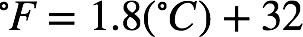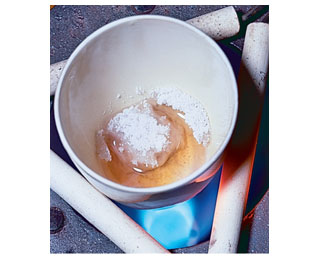# Problem: The melting point of table salt is 1474 oF .What temperature is this on the Celsius scale?

###### FREE Expert Solution

The equation for converting from deg. Celcius (˚C) to deg. Farenheit (˚F) is:We're given the melting point of table salt in ˚F so we can use the equation above to calculate the temperature in ˚C:

79% (389 ratings)###### Problem Details
The melting point of table salt is 1474 oF .What temperature is this on the Celsius scale?